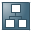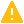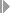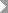Show TOC

###Period-End Closing in Product Cost by Order: ScenarioThe following provides an overview of the functions available for the period-end closing process in the Product Cost by Order component.

#### Prerequisites

The costs were assigned directly to the manufacturing orders.

If manufacturing orders are linked to product cost collectors, these manufacturing orders are ignored in the period-end closing activities of Cost Object Controlling during the processing of the production orders and process orders. The period-end closing process is performed at the level of the product cost collectors in the Product Cost by Period component.

Manufacturing orders are only included in the period-end closing process when the status of the order is not one of the following:

• LKD (Locked)

• CLSD (Closed)

• DLFL (Deletion flag)

• DLT (Deleted)Note

You can significantly improve the performance of the period-end closing transactions by setting the status DLFL for manufacturing orders that meet all of the following conditions:

• Orders that are delivered or technically completed

• Orders for which variances have been calculated

• Orders for which you do not anticipate any further follow-up costs

End of the note.

You can still withdraw the deletion flag after it has been set, for example if follow-up costs are incurred for the order. Deletion indicators, however, cannot be withdrawn.Caution

If you update quantities and values separately in Materials Management (MM), make sure that all quantity movements are valuated before you run the period-end closing transactions.

End of the caution.

#### Process

You can use the Schedule Manager (CA) in the period-end closing process of Product Cost by Order. The Schedule Manager simplifies the period-end closing activities. In Product Cost by Order, you can use the Multilevel Worklist of the Schedule Manager. The multilevel worklist can significantly improve performance and facilitate error correction.

Multilevel worklists enable you to do such things as:

• Display the objects in a processing step sequence that have errorsNote

Each function in the period-end closing process is regarded as a separate processing step. A group of processing steps that are performed one after the other is called a processing step sequence.

End of the note.
• Eliminate the cause of the error, or specify manually by means of the processing status that the system should skip that particular processing step.

• Have the system go through the processing step sequence again (once you have corrected the errors or set the processing statuses that control further processing of the objects)

The system then only processes the objects that had errors and the objects whose processing was forced manually.

The following section contains information on using the Schedule Manager in connection with a multilevel worklist: Starting Transactions/Reports Online, Scheduling Jobs and Job Chains, Worklist

The following functions are available in the period-end closing process in Product Cost by Order:

1. Template allocation

2. Revaluation of activity types and business processes using actual prices

The activity types performed at a cost center are initially valuated with the planned activity price of the activity types. You can revaluate the activity types on the basis of the actual costs charged to the cost center. The difference between the planned activity prices and the actual activity prices is then applied to the cost objects (such as the production orders).

If the application component Actual Costing/Material Ledger is active and you perform actual costing there, you can also charge the difference between the plan activity price and the actual activity price directly to the material. In this case you do not perform the function Revaluation at Actual Prices.

For more information, go into Customizing underControllingProduct Cost ControllingActual Costing/Material LedgerActual CostingActivate Actual Costing.

You calculate percentage or quantity-based overhead rates using overhead calculation on the basis of the actual costs incurred to date.

4. Preliminary settlement for co-products, rework

If you are manufacturing co-products with a manufacturing order, you distribute the actual costs collected on the order header to the order items on the basis of equivalence numbers. You then calculate WIP and variances, and settle. These functions are performed with reference to the order item.

5. WIP calculation

You calculate the work in process for each manufacturing order that has a valid results analysis key.

There are two ways to calculate WIP:

• WIP calculation using the actual costs incurred to date

This method is used in conjunction with cumulative variance calculation and full settlement.

• WIP calculation at target costs

This method is used in conjunction with periodic variance calculation and periodic settlement.Caution

If you want to valuate WIP at target costs, make sure that the routing of the manufacturing order is the same as the routing used to valuate the work in process. If you change the routing and then calculate WIP, the WIP calculation will be faulty.

End of the caution.Note

If you are using a valuated sales order stock, note the special processing requirements in the calculation of WIP for the valuated sales order stock. WIP calculation in this case is based on Standard Price with Valuated Sales Order Stocks, which differs from make-to-stock production.

End of the note.

Once you settle, you can pass the work in process to Financial Accounting (FI) and Profit Center Accounting (CO-PCA).

6. Variance calculation

You can calculate variances by cost element. The system assigns the variances to variance categories (such as input quantity variances and resource-usage variances). This provides you with information on why the actual costs deviated from the planned costs and where you should take corrective action. For a detailed explanation of the variances, use the explanation facility of variance calculation.

The variances are updated to the manufacturing order. If you are using Profitability Analysis (CO-PA), you must calculate the variances in order to settle the price differences to Profitability Analysis. After settlement, you then have the complete actual costs in Profitability Analysis.

Here you calculate variances for each manufacturing order that has a valid variance key.

There are two methods of variance calculation selected through the variance key:

• Cumulative variance calculation

• Periodic variance calculation

If you calculate the variances by period, you can assign the manufacturing orders to cost object hierarchies.

When it calculates the variances, the system uses the planned costs for each operation to valuate the scrap quantities confirmed for the operations. In periodic variance calculation, the system accesses the standard cost estimate for the material, the planned costs of the preliminary costing for the order, or the alternative cost estimate for material according to the valuation variant for WIP and scrap.

In cumulative variance calculation, the scrap is always valuated at standard costs.Caution

Make sure that the routing of the manufacturing order is the same as the routing on which the calculation of scrap variances is based, because the system cannot calculate the scrap if the routing is changed.

End of the caution.

You can view the variances in the information system at any time. The report category Summarized Analysis shows aggregated data, such as at plant level or controlling area level.

When the system calculates the variances, it sets the status VCAL (variances calculated). The system uses this status to select the orders for which variances have been calculated.

This data is taken into account in summarization. You can create summarization hierarchies in the information system to view the cumulative variances by plant or controlling area, for example.

7. Settlement

• Settlement of work in process

When you perform settlement at the end of the period, the system checks whether the manufacturing orders to be settled have a results analysis key. If work in process has been calculated with that results analysis key, the system generates corresponding postings in Financial Accounting (FI) and Profit Center Accounting (EC-PCA).Note

Even if the manufacturing order has the settlement rule FUL (full settlement), the work in process is still settled by period.

End of the note.

The work in process is settled on the basis of posting rules that you define in Customizing for Product Cost Controlling.

• Settlement of the order balance and the variances

For manufacturing orders that have the status delivery completed or technically completed, the difference between the debit and credit of the order (the balance of the order) is transferred to Financial Accounting. Depending on the price control indicator of the material, the balance is settled to a price difference account or a stock account.

If Profit Center Accounting is active, the balance is also passed to that component.

If the Actual Costing/Material Ledger component is active, the balance is also transferred to that component.

In the case of orders with full settlement, the total variance calculated in variance calculation is equal to the balance of the order.

In the case of orders with periodic settlement, the total variance calculated in variance calculation is equal to the balance of the order less the work in process.

When you settle, the total variances calculated with target cost version 0 are normally transferred to Profitability Analysis (CO-PA) broken down into variance categories.Note

The variances settled to CO-PA are normally broken down into variance categories. However, you can also settle the order balance less the work in process to CO-PA.

End of the note.

Settlement is performed on the basis of a settlement rule which the system creates automatically when you create a manufacturing order.Note

If you have assigned the manufacturing orders to a sales order and are using a nonvaluated sales order inventory, then at the end of the period you settle all actual costs to the sales order item. The calculation of work in process at the level of manufacturing orders is unreliable with a nonvaluated sales order inventory and individual requirements materials with multilevel BOMs. The calculation of variances on the manufacturing order is not supported in this case. Instead, you carry out results analysis on the sales order item.

End of the note.

If you have assigned the manufacturing orders to a cost object hierarchy, you perform the activities at the end of the period in the Product Cost by Period component.

For information on the control parameters for Cost Object Controlling, see the following section:

Control Parameters in Cost Object Controlling

For information on variance calculation, refer to the following section:

Variance Calculation

For information on period-end closing with cost object hierarchies, see the following documentation:

Product Cost by Period

For information on period-end closing in the Product Cost by Sales Order component, refer to the following documentation:

Product Cost by Sales Order

For detailed information on displaying the data, refer to the documentation Product Cost Controlling Information System.